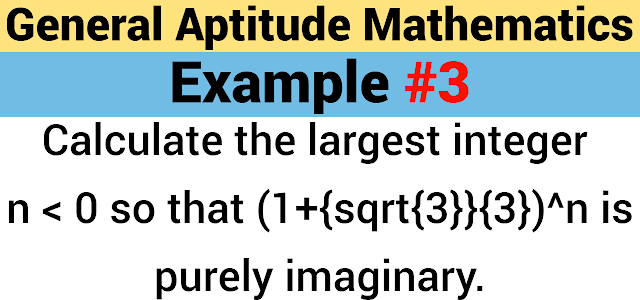# Calculate the largest integer $n < 0$ so that $(1+\frac{\sqrt{3}}{3})^n$ is purely imaginary

##Question:

Calculate the largest integer $n < 0$ so that $(1+\frac{\sqrt{3}}{3})^n$ is purely imaginary.

$(1+\frac{\sqrt{3}}{3})^n$
$=(1+\frac{1}{\sqrt{3}})^n$
$r=\sqrt{1^2+{\frac{1}{\sqrt{3}}}^2}$
$\therefore r=\frac{2}{\sqrt{3}}$
$\theta=tan^{-1}(\frac{\frac{1}{\sqrt{3}}}{1})$
$\therefore \theta=tan^{-1}(\frac{1}{\sqrt{3}})$
$\therefore \theta=\frac{\pi}{6}$
$\therefore(1+\frac{1}{\sqrt{3}})^n=[\frac{2}{\sqrt{3}}(cos\frac{\pi}{6}+isin\frac{\pi}{6})]^n$
$\therefore(1+\frac{1}{\sqrt{3}})^n=(\frac{2}{\sqrt{3}})^n(cos\frac{n\pi}{6}+isin\frac{n\pi}{6})$
Since, the number is purely imaginary,
$\therefore(\frac{2}{\sqrt{3}})^ncos\frac{n\pi}{6}=0$
$\therefore cos\frac{n\pi}{6}=0$
we know that, $cos\theta=0 \implies \theta=(2m-1)\frac{\pi}{2}$
$\therefore \frac{n\pi}{6}=(2m-1)\frac{\pi}{2}$
$\therefore n=3(2m-1)$
Since, $n<0$
$\therefore 3(2m-1)<0 \implies 2m-1<0$
$\implies m<\frac{1}{2}$
Choose, $m=0$
$\therefore n=-3$